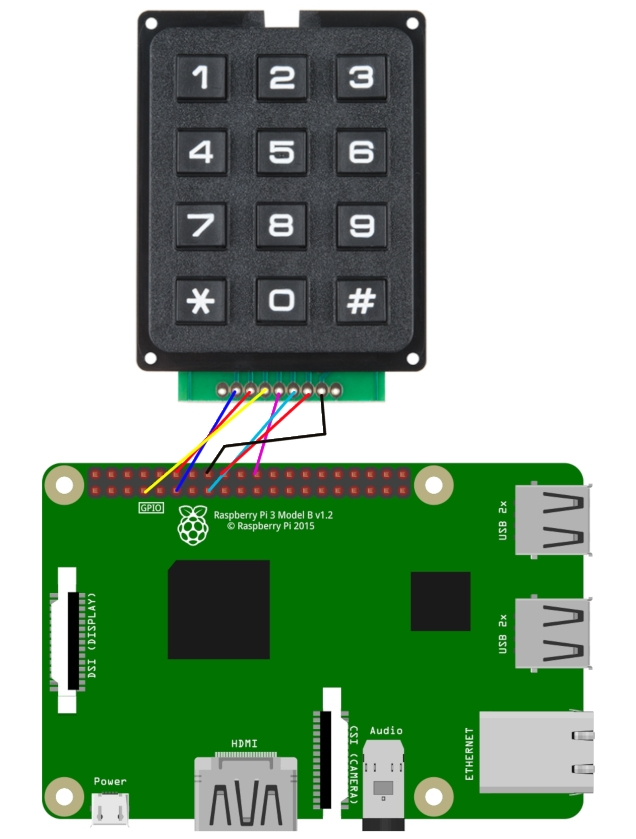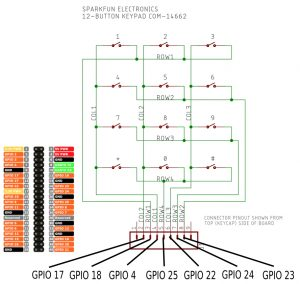Use a Sparkfun COM-14662 12 digit 3*4 matrix keypad with a Raspberry Pi for PIR alarm system

The keypad we used is a Sparkfun Electronics COM14662 which is available from Pimoroni in the UK for £3.50. Similar looking keypads are available on Aliexpress.com https://www.aliexpress.com/item/12-Key-Membrane-Switch-Keypad-4-x-3-Matrix-Array-Matrix-keyboard-membrane-switch-keypad/32824251000.html for \$2.20This next image shows you how the keypad is wired internallyThe examples use the same GPIO pins as Sparkfun Electronics COM14662. If you’re using a different keypad you’ll need to look at the documentation and maybe make changes.

The Python code uses the Raspberry Pi’s internal pull-up resistors, otherwise you’d need a 10k resistor on each col line to stop the GPIO 4/17/22 inputs floating around between 1 and 0.

The software that figures out which button was pressed first scans all the col lines for an input and then switches the GPIO inputs around and scans the row lines instead, to determine exactly which key was pressed. Fortunately there’s some existing Python code to do this for us:

I cut and pasted both examples into a nano editor session and saved them, but they’re also in our demo zip file. You can get that with:

You can run the demo with

and you’ll be able to enter a 4 digit pin and see it printed.

We also built our own working alarm system demo using a PIR movement sensor connected to pins 5V, GND and GPIO8 which you can run with

python alarm2.py

This script lets you input a 4 digit pin. If you enter the correct pin, which is 5678, then the alarm will arm. Once the alarm is armed, any movement detected by the PIR will print a movement detected message to the console. There are other scripts that build on this to email you a photo of the intruder and write the time and date of PIR alerts to a log file.

from time import sleep
import RPi.GPIO as GPIO
import os

digitCount = 0
pin = [0,0,0,0]
finalPin = "0000"
validPin = "5678"
armState = 0
pirPin = 8

GPIO.setmode(GPIO.BCM)
GPIO.setwarnings(False)
GPIO.setup(pirPin, GPIO.IN)

def digitreturn():
# Loop while waiting for a keypress
r = None
while r == None:
r = kp.getKey()
pirtest()
return r

def message():
os.system('clear')

def pirtest():
if GPIO.input(pirPin) and armState == 1:
print("Movement Detected. Intruders!")
sleep(3)
message()

while True:
digit = digitreturn()
print digit
pin[digitCount] = digit
digitCount = digitCount + 1
sleep(0.25)
if digitCount == 4:
finalPin = (str(pin) + str(pin) + str(pin) + str(pin))
print(finalPin)
if validPin == finalPin:
print("You entered the correct PIN")
if armState == 0:
print("alarm will arm in 5 seconds, get out now")
sleep(5)
print("armed")
armState = 1
sleep(1)
message()
elif armState == 1:
print("alarm is now disarmed")
armState = 0
digitCount = 0

and this version will take a time and date stamped photo of the intruder for you

import picamera
from time import sleep
import RPi.GPIO as GPIO
import os
import datetime

digitCount = 0
pin = [0,0,0,0]
finalPin = "0000"
validPin = "5678"
armState = 0
pirPin = 8
camera = picamera.PiCamera()

GPIO.setmode(GPIO.BCM)
GPIO.setwarnings(False)
GPIO.setup(pirPin, GPIO.IN)

def digitreturn():
# Loop while waiting for a keypress
r = None
while r == None:
r = kp.getKey()
pirtest()
return r

def message():
os.system('clear')

def pirtest():
if GPIO.input(pirPin) and armState == 1:
now = datetime.datetime.now().strftime("%y-%m-%d--%H-%M-%S")
print("Movement Detected " + now + ".  Taking a photo")
camera.start_preview()
sleep(3)
camera.annotate_background = picamera.Color('black')
camera.annotate_text = datetime.datetime.now().strftime('%Y-%m-%d %H:%M:%S')
camera.capture('/home/pi/' + now + 'image.jpg')
camera.stop_preview()

message()

while True:
digit = digitreturn()
print digit
pin[digitCount] = digit
digitCount = digitCount + 1
sleep(0.25)
if digitCount == 4:
finalPin = (str(pin) + str(pin) + str(pin) + str(pin))
print(finalPin)
if validPin == finalPin:
print("You entered the correct PIN")
if armState == 0:
print("alarm will arm in 5 seconds, get out now")
sleep(5)
print("armed")
armState = 1
sleep(1)
message()
elif armState == 1:
print("alarm is now disarmed")
armState = 0
digitCount = 0

This version will send you a photo of the intruder as an email attachment

import picamera
from time import sleep
import RPi.GPIO as GPIO
import os
import datetime

#email setup below
from email.MIMEMultipart import MIMEMultipart
from email.MIMEText import MIMEText
from email.MIMEImage import MIMEImage
import smtplib

digitCount = 0
pin = [0,0,0,0]
finalPin = "0000"
validPin = "5678"
armState = 0
pirPin = 8
camera = picamera.PiCamera()

GPIO.setmode(GPIO.BCM)
GPIO.setwarnings(False)
GPIO.setup(pirPin, GPIO.IN)

def digitreturn():
# Loop while waiting for a keypress
r = None
while r == None:
r = kp.getKey()
pirtest()
return r

def message():
os.system('clear')

def pirtest():
if GPIO.input(pirPin) and armState == 1:
now = datetime.datetime.now().strftime("%y-%m-%d--%H-%M-%S")
print("Movement Detected " + now + ".  Taking a photo")
camera.start_preview()
sleep(3)
camera.annotate_background = picamera.Color('black')
camera.annotate_text = datetime.datetime.now().strftime('%Y-%m-%d %H:%M:%S')
camera.capture('/home/pi/' + now + 'image.jpg')
camera.stop_preview()
# Send an email section. Make a new gmail account just for your Pi to use.  Then enable "less secure apps"
msg = MIMEMultipart()
mailer = smtplib.SMTP('smtp.gmail.com:587')
mailer.starttls()
# Recipient is the regular email account on your phone or PC.
mailer.sendmail("myraspberrypi@gmail.com", ["recipient@gmail.com"], msg.as_string())
mailer.close()

message()

while True:
digit = digitreturn()
print digit
pin[digitCount] = digit
digitCount = digitCount + 1
sleep(0.25)
if digitCount == 4:
finalPin = (str(pin) + str(pin) + str(pin) + str(pin))
print(finalPin)
if validPin == finalPin:
print("You entered the correct PIN")
if armState == 0:
print("alarm will arm in 5 seconds, get out now")
sleep(5)
print("armed")
armState = 1
sleep(1)
message()
elif armState == 1:
print("alarm is now disarmed")
armState = 0
digitCount = 0

Sorry, the comment form is closed at this time.